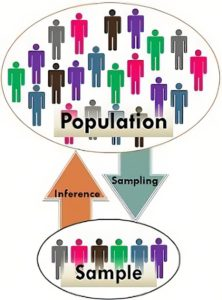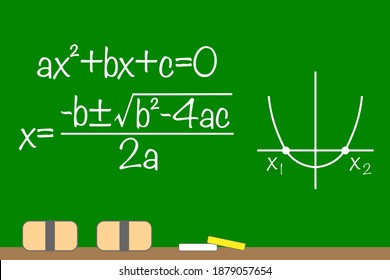## MS Math April 25-29, 20226th Grade Math – Mrs. Evans

This week we will finish Chapter 10.   We will be able to calculate the area and perimeter of most simple forms – squares, rectangles, parallelograms, triangles, trapezoids and regular polygons.  We can use these simple shapes to model composite shapes in order to find the area of these figures.  By knowing how to find the area of simple shapes we can use our critical thinking to apply it to many shapes we see.  Here is a video showing how to apply simple shape formula to find the area of composite shapes to find the area and the perimeter:

Math Grade Math – Mrs. Evans and Mrs. VonFeldt

This week, the 7th graders will be finishing Module 10. In Module 10, we have understood that when we want to study a population of something, we can study a random sample of that population, evaluate the data, and make inferences (predictions or educated guesses).  This is very useful in the real world.  This is an overall picture of how the process works:Algebra – Mrs. VonFeldt

This week we will learn the last two strategies for solving quadratic equations: Completing the Square and the Quadratic Formula. The Quadratic Formula can be used for any quadratic equation, however, it is particularly useful when the “a” “b” and “c” terms are not integers. It is so useful in fact, that it is worth spending the time to memorize it. (see the song below). We will spend time this week reviewing all the strategies we have learned and determining which one is the best for a particular equation. There will be a test on Chapter 9 next week.

QUADRATIC EQUATION:## MS Math April 18-22, 20226th Grade Math – Mrs. Evans

This week we will continue studying geometry in Chapter 10.  We have been investigating shape areas and creating formulas that we can use to calculate area.  We have already found the formula for squares, rectangles, parallelograms and triangles.  This week we will find formulas for trapezoids and regular polygons.  We will use these formulas to find the area of composite figures, which are figures that are made up of more than one simpler shape.

Math Grade Math – Mrs. Evans and Mrs. VonFeldt

The 7th graders are studying statistics, working lessons in Module 10.  We are looking at populations of things we are interested in studying by sampling them.  We want to have random, unbiased samples surveyed.  If we have this, we can assume that we can make reasonable inferences (predictions) about the population.  We are learning to plot data so that we can make predictions.  We are using dot plots and box plots.  Dot plots are easy to create while box plots are a little more complicated.  Here is a video showing how to create these.

8th Grade Math – Mrs. Ernest

The 8th graders will be practicing solving multistep equations. To work through these problems, students will first need to copy down the problem, then circle and box different terms.